Ex 14.2

Chapter 14 Class 7 Symmetry
Serial order wise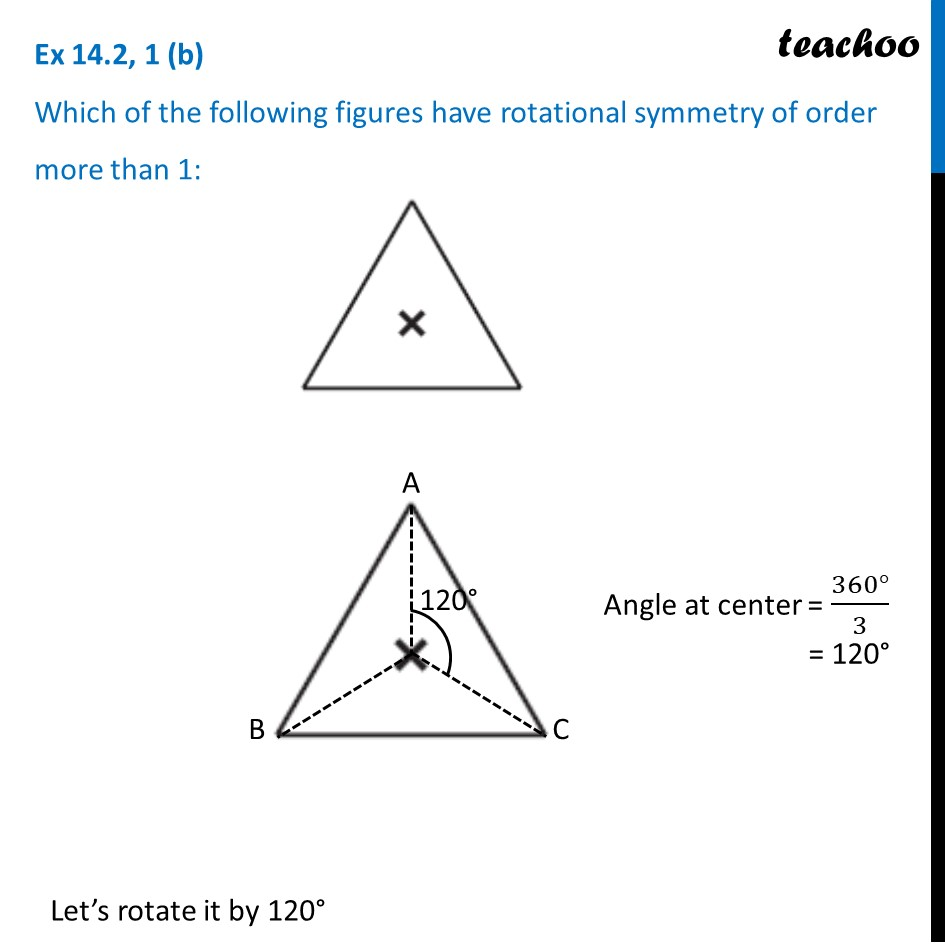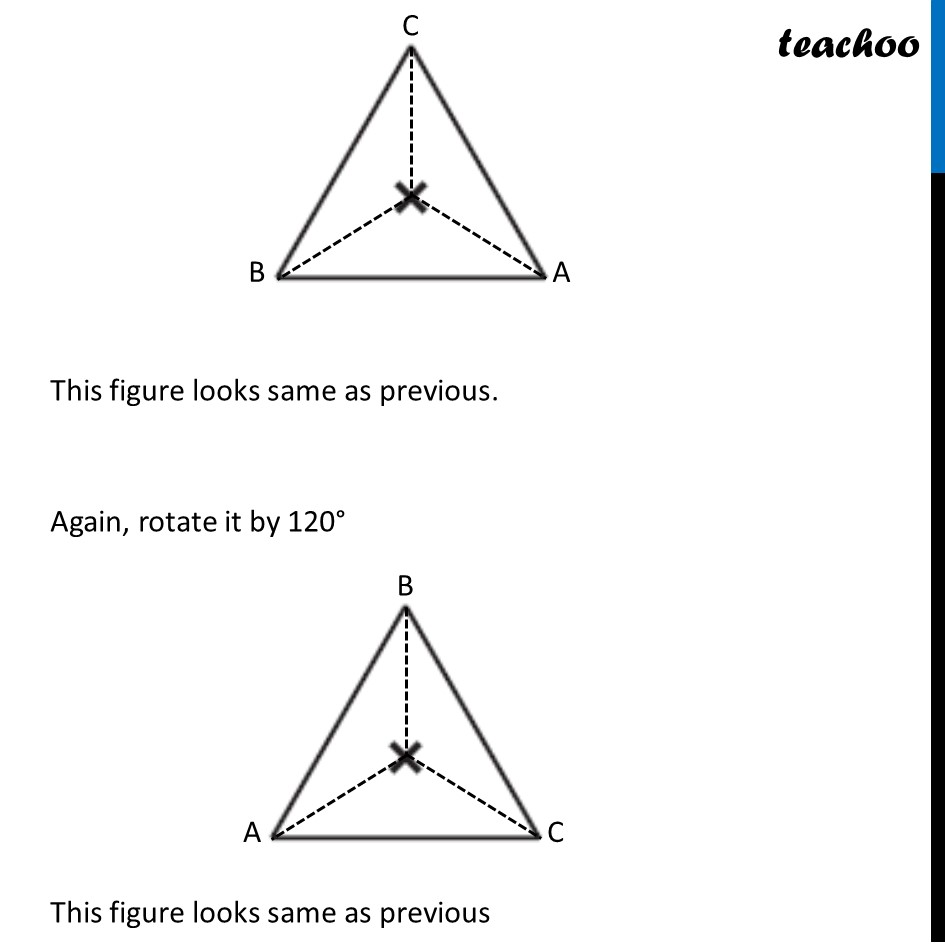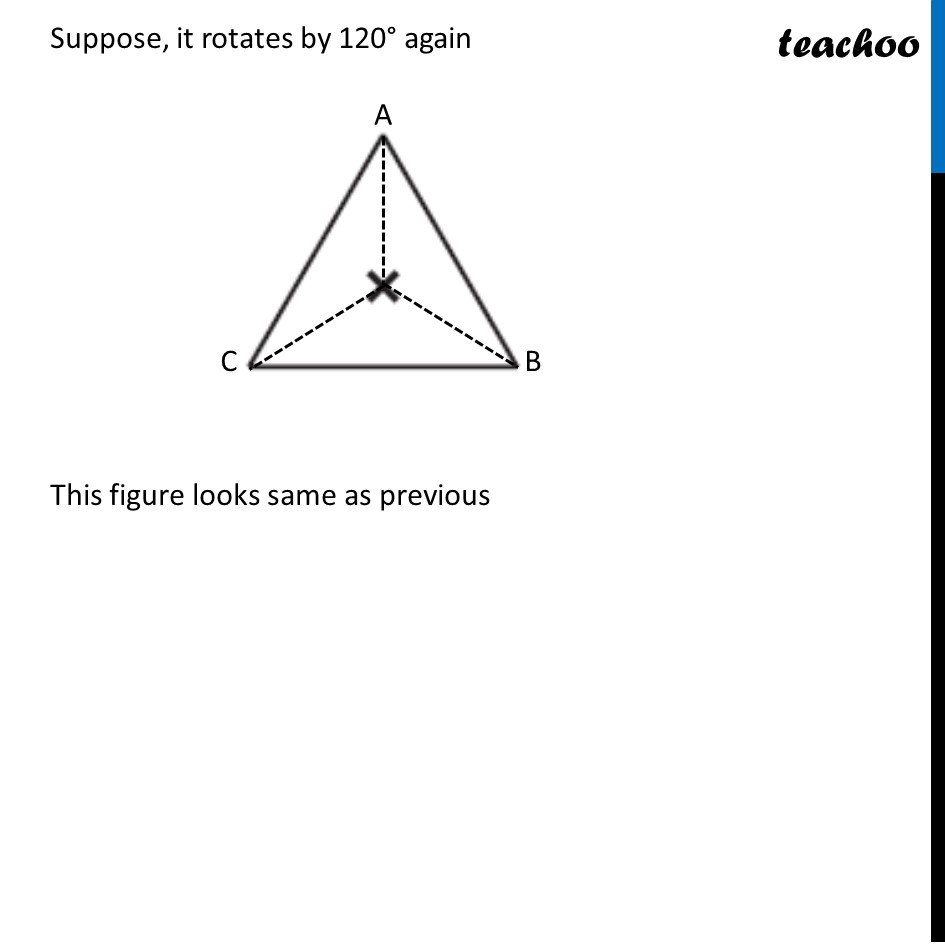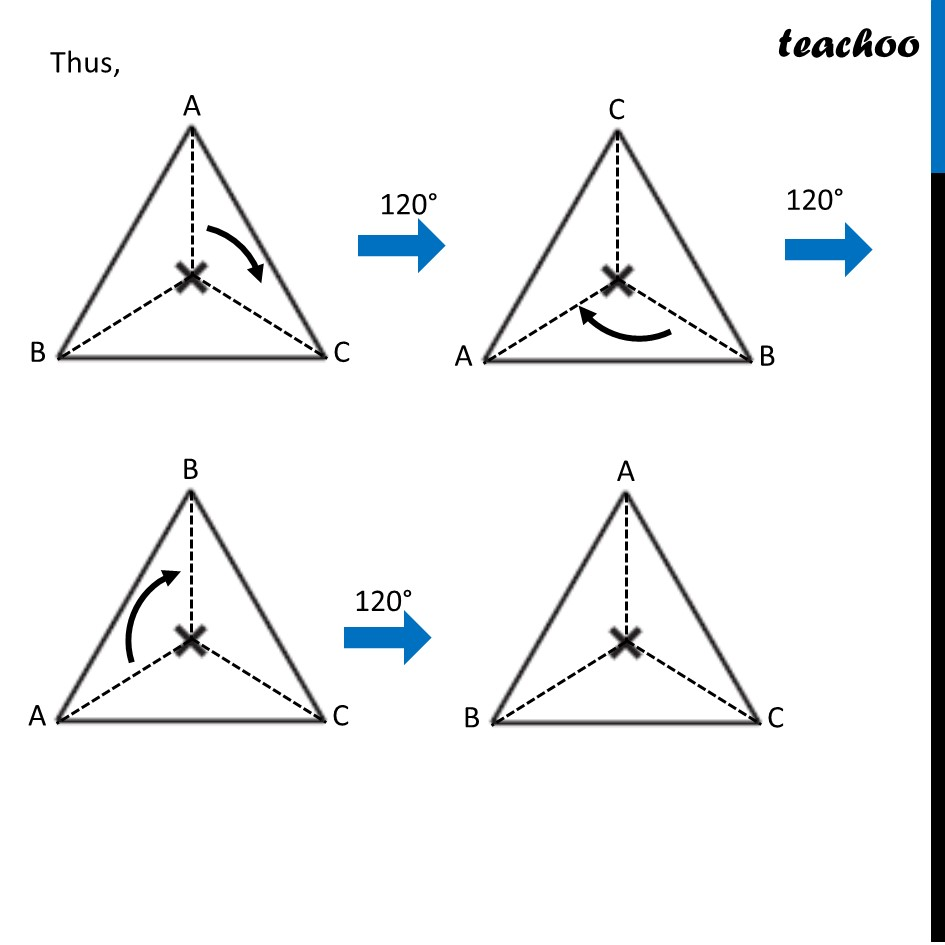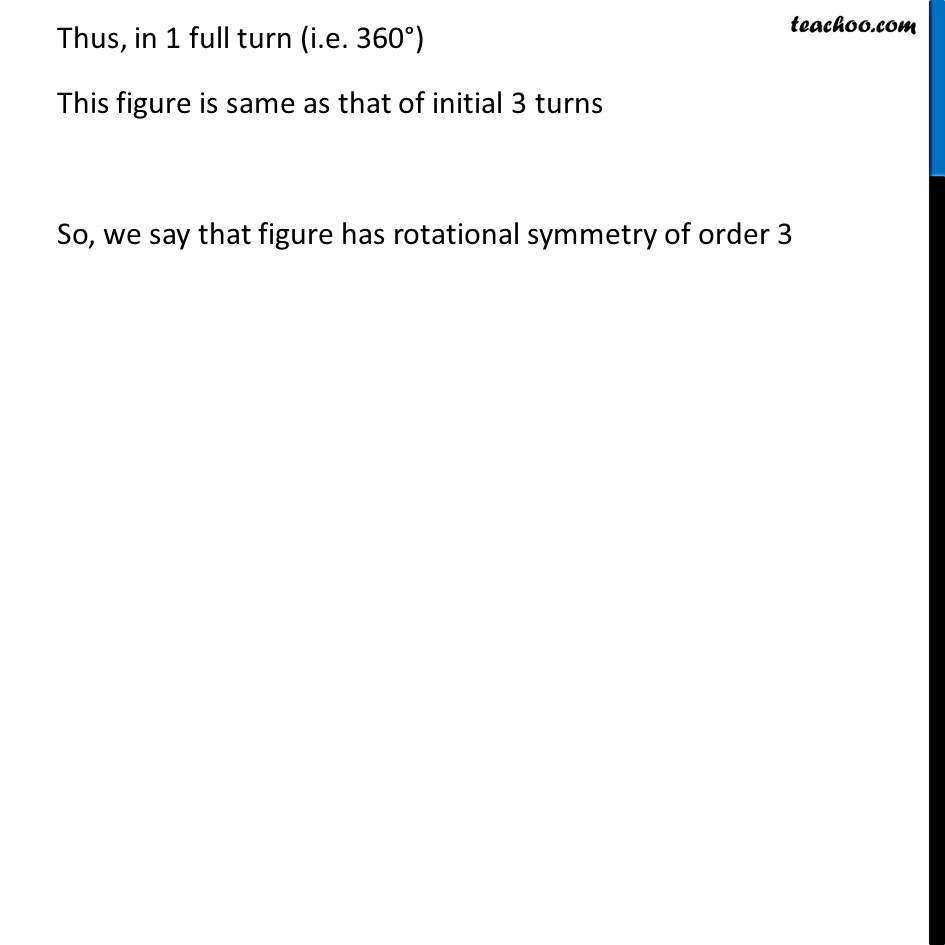### Transcript

Ex 14.2, 1 (b) Which of the following figures have rotational symmetry of order more than 1: Angle at center = (360°)/3 = 120° Let’s rotate it by 120° This figure looks same as previous. Again, rotate it by 120° This figure looks same as previous Suppose, it rotates by 120° again This figure looks same as previous Suppose, it rotates by 120° again This figure looks same as previous Thus, Thus, in 1 full turn (i.e. 360°) This figure is same as that of initial 3 turns So, we say that figure has rotational symmetry of order 3